# Logic: Not & And

Statements by themselves aren't that interesting; let's see how to combine them.

### "not"

A writing teacher once told me the best way to clean up your writing is to eliminate as many adverbs and adjectives as possible. But one very important adverb--which changes the meaning of anything it touches--is the word not. Let's formalize this.

Definition. Given a statement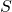, the statement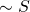(read It is not the case thator the abbreviated version notis true exactly whenis false.

Note that ifis a statement, it's either true or false; in either case, we know what truth valuehas. Sois by definition a statement. Also note the word exactly, which modifies when.

Proposition 1.is true exactly whenis true.

Proof. There are only two kinds of statements, namely true and false.

Ifis false, thenis true (this is the definition of). But the only (that's the exactly in the definition of) way forto be true would be ifwere false; sinceis true, we knowis false. So in this situation,andare both false.

Ifis true, thenis false (remember,is only true whenis false). Sois true. So here again,andagree with one another.

In both cases, we've seen thatandagree. Thus whenis true,is true; and this is the only way forto be true.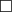.

Notice thatis a different statement from. For example, ifis I wear a plaid shirt every day, thenis It is not the case that I wear a plaid shirt every day andis It is not the case that it is not the case that I wear a plaid shirt every day.

But of courseandare the same insofar as truth goes. One might say they mean the same thing.

To make better formal sense of the distinction between being the same statement and merely being statements that mean the same thing, we need the following definition:

Definition. A propositional formula or propositional form is a string of logical connectives and variables, so that when each of the variables is substituted with a statement, the result is a statement.

For example,is a propositional formula because if we supply a statement in place of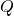, we obtain a statement.

Definition. Two propositional forms are equivalent if, whenever we substitute the same statements in for their variables, we obtain statements with the same truth values.

Armed with this definition, we can rewrite Proposition 1 in a different way:

Restatement of Proposition 1.and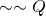are equivalent.

### denials, useful and otherwise

Definition. A formula equivalent tois called a denial

Observe that we've just used the word "denial" to mean two different, but related, things: there are denials of formulae, and denials of statements. One should be careful to keep track of subtle differences like this, which happen so often most mathematicians eventually just learn not to notice them anymore.

of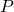. If we plug a statement in to the variable, we obtain a denial of that statement.

E.g.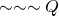and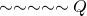are denials of.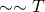is a denial of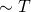.

E.g. A denial of Andrew Cooper is from Texas is It is not the case that Andrew Cooper is from  Texas.
Another denial is Andrew Cooper is not from Texas.
Another denial is Andrew Cooper is either from someplace other than Texas, or he is not from anyplace in particular, a drifter if you will, rootless, without a home.

These examples show that there are many different denials of any given formula or statement.

One question I want you to ask, and to keep asking is: is a given formulation of a statement useful to me? Utility is in the eye of the beholder, so I won't give you a formal definition of what I mean when I say useful denial. But I'll say this: merely attaching It is not the case that... to the beginning of a statement probably will not yield any insight.

You might be asking yourself: why should I expect that formulating a denial would yield any insight, anyway? Here's why, and it's a very important less in thinking that I hope you take away from this course into the rest of your life beyond mathematics. Statements are, by definition, either true or false (and not both). So, when we are faced with a statement we don't understand (or wish to understand better, or wish to prove), we usually ask questions like

• What does this statement mean?
• If this statement were true, what else would be true?
• When have I encountered similar (or just similar-looking) statements in the past?

These are great questions, but they don't always get us where we need to go. Sometimes they can even lead us astray. Since we know that the statement in question is either true or false, we could as well ask about it:

• What would it mean for this statement to be false?

That is, we could formulate a denial of the statement. If we articulate all the ways the statement could be false, then we have also articulated what it means for the statement to be true: that's just everything not on our list.

I call this method what-if-not thinking, and we're going to use it a lot. But it depends, critically, on being able to formulate denials precisely and accurately. (Don't worry, we'll develop lots of practice.)

### truth tables

Definitions like that ofare kind of hard to read, so let's record them symbolically. We can think ofas being like a function: you tell it the truth value of, and it tells you the truth value of. So, just like you first did in some long-ago algebra class, we can record the values in a table. We call such a table a truth table. The truth table forlooks like this:T F F T

We can make a truth table for any formula; here's the truth table for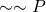:T T F F

Observe that we always list the input values (the first column) in the same order.

It's pretty clear that Two formulae are equivalent if the output columns of their truth tables are the same.

### "and"

not was nice, but it only acts on one statement or formula. (We call such operations unary, from the Latin unus "one".) Let's get some binary operations: operations which combine two statements or formulae. We'll start with the word and.

Before reading any further, make sure you've done the preticipation exercise: read the dictionary.com entry on the word and and see if it would help you understand what the word meant, if you didn't already know. Try this definition also.

Instead of giving a wordy definition, I'll just give the truth table for "and". The symbol we use is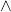. Since there are two inputs, each of which has two possible truth values, there are four possible inputs: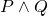T T T 1 T F F 2 F T F 3 F F F 4

Because we're going to use this table rather than just look at it, I've given numbers to the rows.

Let's play around a bit with this. What's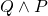? We'll make a truth table. In order to compare it with the truth table for, we need to list the inputs in the same order.T T T 1 T F F 3 F T F 2 F F F 4

Look carefully at the row labels. In order to populate row 2 of thetable, we have to look at row 3 of thetable -- this row is the "FALSE and TRUE" row.

We can conclude from this truth table that

Proposition.andare equivalent formulae.

Or, somewhat more pithily, we say

Restatement of Proposition.is commutative.

While this proposition may not surprise you, it does indicate a slight difference from the  ordinary-English word and.

Let's try something else out, too: what about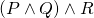? This time we're have three inputs, each of which has two possible truth values, for a total of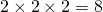possible input truth values: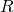T T T T T 1 F F 2 F T F F 3 F F 4 F T T F F 3 F F 4 F T F F 3 F F 4

Here I've done two tricks: first, instead of writing so many Ts and Fs, I combined some rows in theandcolumns to make the table easier to read. Second, I wrote down the intermediate step of computingas a separate column. The last column records which row of the truth table forI used to go combine the values in the third and fourth columns to get the truth value in the last column.

Exercise. Make a truth table for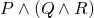.

Proposition.is equivalent to.

The pithy way to say this is

Restatement of Proposition.is associative.

Finally, let's do two truth table computations withand:

Exercise. Make truth tables for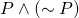and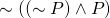. Before beginning this, think hard about how many rows and how many columns your truth table will require. Then see if you notice anything interesting.

The result of the previous exercise will leave us with the need for the following definition:

Definition. A formula which always has a truth value of T, no matter what inputs it takes, is called a tautology. A formula which always has a truth value of F, no matter what inputs it takes, is called a contradiction.

If we're thinking of propositional formulae as functions, then tautologies and contradictions are the constants. (There are only two constant functions, because statements can only be true or false (and not both).)

The philosopher's version of this definition goes something like A tautology is a sentence that is true in virtue of its form. and A contradiction is a sentence that is false in virtue of its form., the point being that neither a tautology nor a contradiction can contain much in the way of meaningful content, because its form gets in the way. But that's all a bit above our heads.

Here's a shocking claim:

and and but mean the same thing.

What do I mean by this? Consider the two statements:

1. I have a dog and he weighs twelve pounds.
2. I have a dog but he weighs twelve pounds.

In terms of the facts on the ground, what do we know in situation 1? That I have a dog. That the dog weighs twelve pounds. What do we know if situation 2? Exactly the same information!

So what's different? Presumably, if I'm saying statement 2, it's because I'm vaguely embarrassed to have such a small dog (sorry, Mugzy). That is, the difference between and and but lies in how the speaker feels about the circumstances. And because that's not something that can be true or false, our logical framework just doesn't care about it.

You might think this is a bug, but I see it as a feature. (Perhaps better to say: you might see this as a bug, and I see it as a feature.) It will allow us to subtly influence our reader's perception -- and our own perception -- in ways that may make our written proofs clearer and easier to understand.

A neat thinking trick you can deploy to help make sure you aren't being unduly influenced (say, when you're watching talking heads): every time someone says but, just repeat what they said with an and instead. And make sure you don't use but when you mean and.